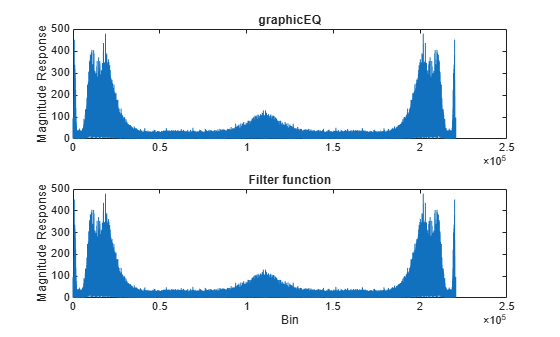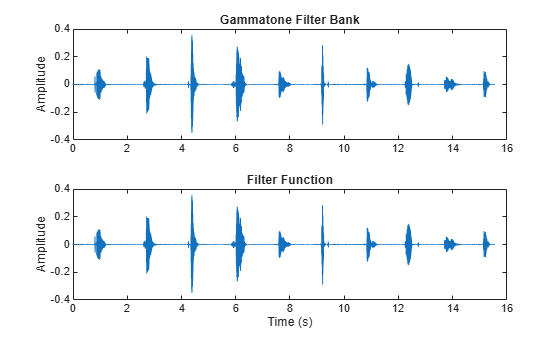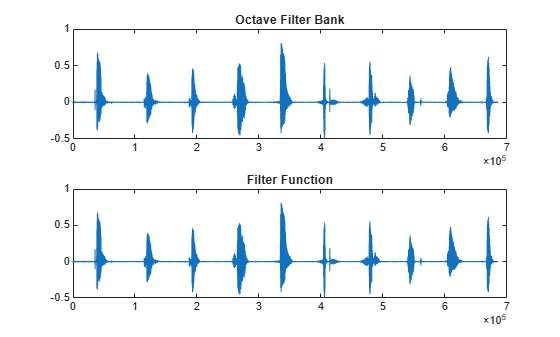Main Content

# coeffs

Get filter coefficients

## Syntax

``[B,A] = coeffs(obj)``

## Description

example

````[B,A] = coeffs(obj)` returns the coefficients of the filters created by `obj`.```

## Examples

collapse all

Cascade Structure

Create a `graphicEQ` and then call `coeffs` to get its coefficients. The coefficients are returned as second-order sections. The dimensions of `B` are 3-by-(`M` * `EQOrder` / 2), where `M` is the number of bandpass equalizers. The dimensions of `A` are 2-by-(`M` * `EQOrder` / 2). The leading unity coefficient is not returned.

```fs = 44.1e3; x = 0.1*randn(fs*5,1); equalizer = graphicEQ('SampleRate',fs, ... 'Gains',[-10,-10,10,10,-10,-10,10,10,-10,-10], ... 'EQOrder',2); [B,A] = coeffs(equalizer);```

Compare the output of the `filter` function using coefficients `B` and `A` with the output of `graphicEQ`. For simplicity, compare output from channel five only.

```channelToCompare = 5; y = x; for section = 1:equalizer.EQOrder/2 for i = 1:numel(equalizer.Gains) y = filter(B(:,i*section),[1;A(:,i*section)],y); end end audioOut_filter = y; audioOut = equalizer(x); subplot(2,1,1) plot(abs(fft(audioOut))) title('graphicEQ') ylabel('Magnitude Response') subplot(2,1,2) plot(abs(fft(audioOut_filter))) title('Filter function') xlabel('Bin') ylabel('Magnitude Response')```Create the default `gammatoneFilterBank`, and then call `coeffs` to get its coefficients. Each gammatone filter is an eighth-order IIR filter composed of a cascade of four second-order sections. The size of `B` is 4-by-3-by-`NumFilters`. The size of `A` is 4-by-2-by-`NumFilters`.

```[audioIn,fs] = audioread('Counting-16-44p1-mono-15secs.wav'); gammaFiltBank = gammatoneFilterBank('SampleRate',fs); [B,A] = coeffs(gammaFiltBank);```

Compare the output of the `filter` function using coefficients `B` and `A` with the output of `gammaFiltBank`. For simplicity, compare output from channel eight only.

```channelToCompare = 8; y1 = filter(B(1,:,channelToCompare),[1,A(1,:,channelToCompare)],audioIn); y2 = filter(B(2,:,channelToCompare),[1,A(2,:,channelToCompare)],y1); y3 = filter(B(3,:,channelToCompare),[1,A(3,:,channelToCompare)],y2); audioOut_filter = filter(B(4,:,channelToCompare),[1,A(4,:,channelToCompare)],y3); audioOut = gammaFiltBank(audioIn); t = (0:(size(audioOut,1)-1))'/fs; subplot(2,1,1) plot(t,audioOut(:,channelToCompare)) title('Gammatone Filter Bank') ylabel('Amplitude') subplot(2,1,2) plot(t,audioOut_filter) title('Filter Function') xlabel('Time (s)') ylabel('Amplitude')```Create the default `octaveFilterBank`, and then call `coeffs` to get its coefficients. The coefficients are returned as second-order sections. The dimensions of B and A are T-by-3-by-M, where T is the number of sections and M is the number of filters.

```[audioIn,fs] = audioread('Counting-16-44p1-mono-15secs.wav'); octFiltBank = octaveFilterBank('SampleRate',fs); [B,A] = coeffs(octFiltBank);```

Compare the output of the `filter` function using coefficients `B` and `A` with the output of `octaveFilterBank`. For simplicity, compare output from channel five only.

```channelToCompare = 5; audioOut_filter = filter(B(1,:,channelToCompare),A(1,:,channelToCompare),audioIn); audioOut = octFiltBank(audioIn); subplot(2,1,1) plot(audioOut(:,channelToCompare)) title('Octave Filter Bank') subplot(2,1,2) plot(audioOut_filter) title('Filter Function')```## Input Arguments

collapse all

Object to get filter coefficients from, specified as an object of `gammatoneFilterBank`, `octaveFilterBank`, or `graphicEQ`.

## Output Arguments

collapse all

Numerator filter coefficients, returned as a 2-D matrix or 3-D array, depending on `obj`.

Data Types: `single` | `double`

Numerator filter coefficients, returned as a 2-D matrix or 3-D array, depending on `obj`.

Data Types: `single` | `double`

## Compatibility Considerations

expand all

Behavior changed in R2020b

## See Also

Introduced in R2019a

## SupportGet trial now